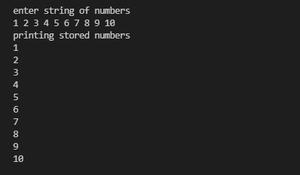Open in App
Not now

# How to Take Input From User Separated By Space in Java ?

• Difficulty Level : Easy
• Last Updated : 15 Sep, 2021

There are 2 methods to take input from the user which are separated by space which are as follows:

1. Using BufferedReader Class and then splitting and parsing each value
2. Using nextInt( ) method of Scanner class

Let us discuss both the methods one by one in order to get a better understanding by implementing the same clean java programs.

Method 1:

Using BufferedReader Class and then splitting and parsing each value.

Procedure:

2. Split this String for str.split(” “)
3. Iterate over the above array and parse each integer value using Integer.parseInt( ).

Example

## Java

 `// Java Program to Take Input from User Separated by Space``// Using BufferedReader class` `// Importing required classes``import` `java.io.BufferedReader;``import` `java.io.IOException;``import` `java.io.InputStreamReader;` `// Main class``// BufferedReaderTest``class` `GFG {` `    ``// Main driver method``    ``public` `static` `void` `main(String[] args)``        ``throws` `IOException``    ``{` `        ``// Creating an object of BufferedReader class``        ``BufferedReader bi = ``new` `BufferedReader(``            ``new` `InputStreamReader(System.in));` `        ``// Custom integer array of size 10``        ``int` `num[] = ``new` `int``[``10``];``        ``// Array of string type to store input``        ``String[] strNums;` `        ``// Display message``        ``System.out.println(``"enter string of numbers"``);` `        ``// Reading input a string``        ``strNums = bi.readLine().split(``" "``);` `        ``for` `(``int` `i = ``0``; i < strNums.length; i++) {``            ``num[i] = Integer.parseInt(strNums[i]);``        ``}` `        ``// Display message``        ``System.out.println(``"printing stored numbers "``);` `        ``// Printing the stored numbers using for loop``        ``for` `(``int` `i = ``0``; i < strNums.length; i++) {``            ``System.out.println(num[i]);``        ``}``    ``}``}`

Output:FIG = OUTPUT OF METHOD 1

Method 2: Using nextInt() method of Scanner class.

Procedure:

1. Using the nextInt() method of Scanner class and scan to scan the input.
2. Using for loop to store input in an array.
3. Iterate through the above array and parse each integer using sc.nextInt()

Example

## Java

 `// Java Program to Take Input from User Separated by Space``// Using Scanner class` `// Importing required classes``import` `java.io.IOException;``import` `java.util.Scanner;` `// Main class``// Scanner class``class` `GFG {` `    ``// Main driver method``    ``public` `static` `void` `main(String[] args)``        ``throws` `IOException``    ``{` `        ``// Display message for better readability``        ``System.out.println(``"enter input "``);` `        ``// Creating an object of Scanner class``        ``Scanner sc = ``new` `Scanner(System.in);` `        ``// Declaring and initializing an array of size 10``        ``int``[] nums = ``new` `int``[``10``];``        ``int` `i;` `        ``// Loop to store input values in nums array``        ``for` `(i = ``0``; i < nums.length; i++) {``            ``nums[i] = sc.nextInt();``        ``}` `        ``//  Display message``        ``System.out.println(``"printing stored values"``);` `        ``// Printing stored values``        ``for` `(i = ``0``; i < nums.length; i++) {``            ``System.out.println(nums[i] + ``" "``);``        ``}``    ``}``}`

Output:FIG = OUTPUT OF METHOD 2

Note: The first method using Bufferedreader class and then splitting and parsing each value is much faster than using nixing() method of Scanner class. It is nearly 2 times faster than the second one. Bel;ow we do provide in order how to calculate the time consume by both methods by using  nanotime method

```// Initializing variables
long startTime, endTime;

// Start time
startTime = System.nanoTime(); {

// Insert code here
// Method 1 or method 2 code
}

// End time
endTime = System.nanoTime();```

My Personal Notes arrow_drop_up